# BitXOr

## BitXOr(x, I)

Returns the bitwise exclusive-OR (XOR) of the integer portions of an array of numbers. Each bit in the result is on only if the corresponding bit is on in an odd number of integers in «x» along index «I». «I» can be omitted when taking the bitwise XOR across the Implicit dimension.

Each integer may have up to 64 bits.

## Examples

To find the bitwise XOR of three numbers, the numbers can be listed in brackets (the implicit index) and the index parameter omitted.

BitXOr([23, 29, 25]) → 19

This is more obvious when we write these same numbers in binary notation:

BitXOr([0b10111, 0b11101, 0b11001]) → 19 { note: 19 = 0b10011 }

Suppose x is the following 2-D array of integers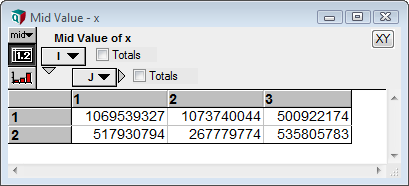which is shown here in binary notation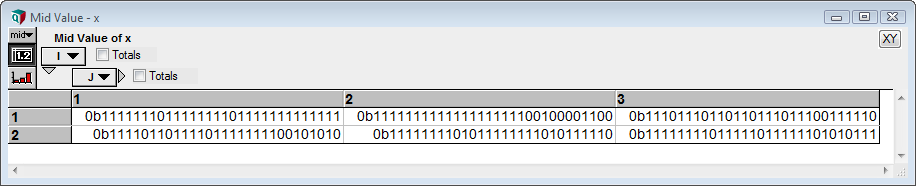then

BitXOr(x, I) →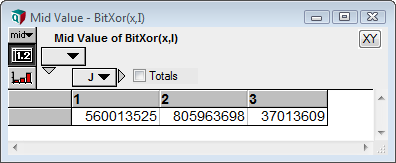=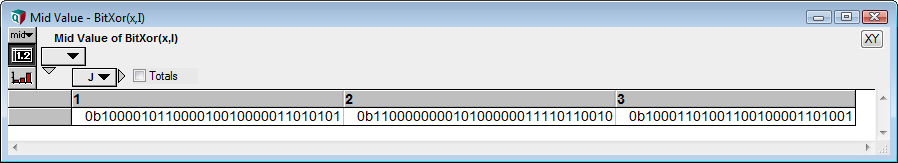BitXOr(x, J) →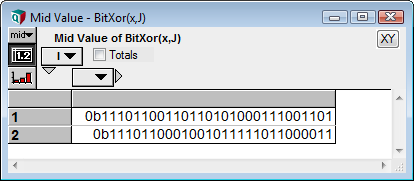## History

Introduced in Analytica 5.0.